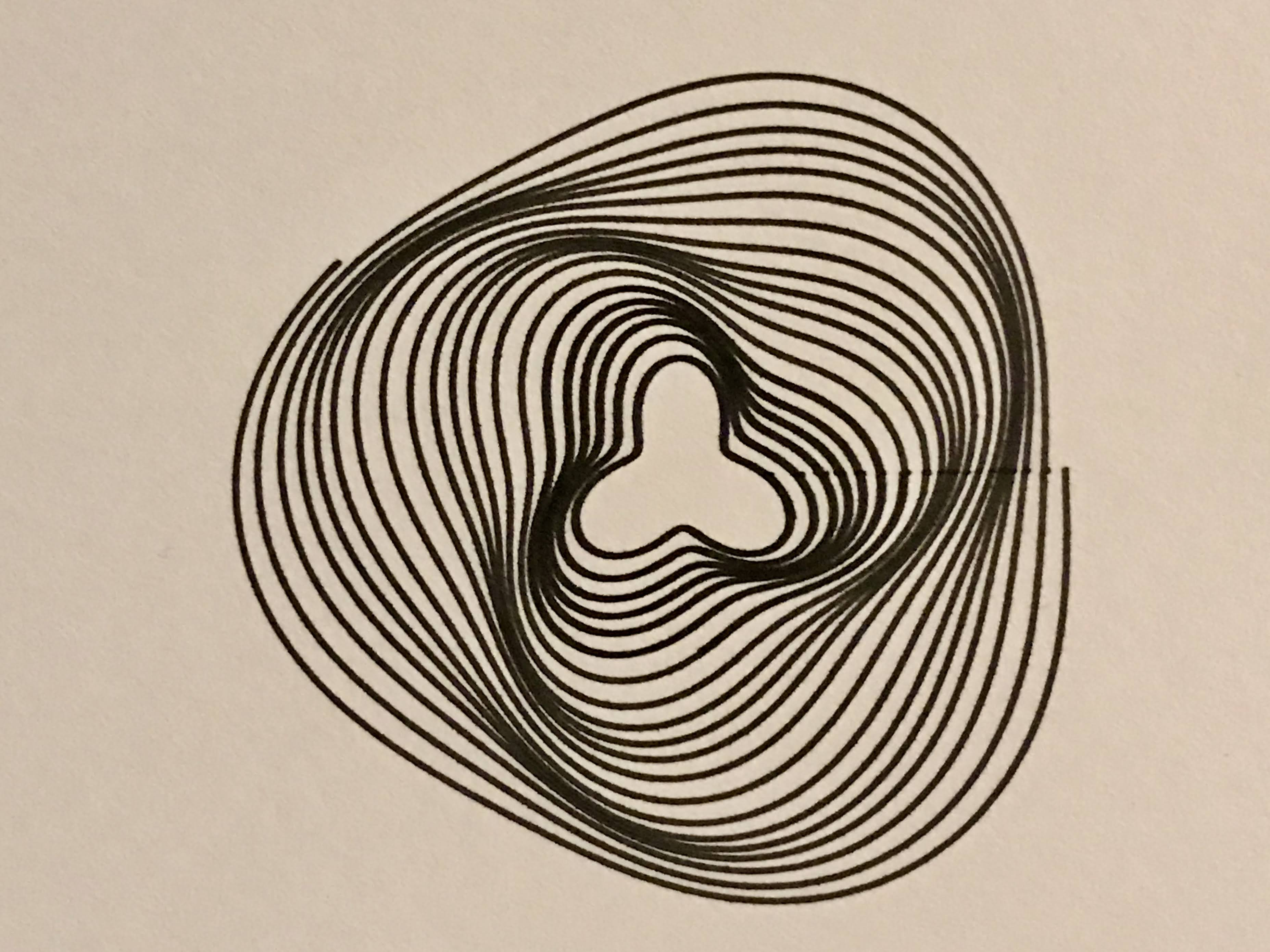## Out, damned spot! A prelude

· [ plotting, out-damned-spot ] · @pentronik

It all started with some little dark dots. See the photograph below. They happened to be in a line, and as such, they were very obvious. What follows is (planned to be) a series of blog posts about my unravelling of the trouble I got into trying to solve this issue. Assuming that I do solve this issue to my own satisfaction. The reason for this to be a series of posts is that I’ll try to keep each post focussed. To see them all together, see the #out-damned-spot tag page. You could think of it like a logbook, but I don’t promise to not edit it for clarity as I understand more.The small marks are from overwriting the same point. Stopping this artifact from appearing in my works has been quite a bit more tricky than I imagined it would be.

In this post, I will describe the original issue, and document as best as I can recollect all the other things that were going on. Luckily I can verify most of this if I desire or need to.1

1. Frame of mind: I wanted a bit more speed after my experience with Series002 postcards. The problem there was the text, but I was starting to explore other aspects of increasing speed. See also the first post about faster circles.
2. I’ve been meaning to add a bit more instrumentation to my code, so there is that. To track number of moves and line segments, pen up and pen down actuations, length of lines and moves.
3. Things that I have changed since: trying out setting const_speed, raising the pen_pos_down value, upping the speed_pendown a bit.
4. Working up to Series003 postcards, so searching for something nice to put on them. See the picture above for the idea that I’ve settled on. But they’ve got to be perfect.

So the basic form of these things for Series003 is

$\begin{eqnarray} x(n) &=& A \cos\left(2 \pi \frac{n}{N}\right) + B \cos\left(m 2 \pi \frac{n}{N} + \phi\right)\\ y(n) &=& A \sin\left(2 \pi \frac{n}{N}\right) + B \sin\left(m 2 \pi \frac{n}{N} + \phi\right).\end{eqnarray}$

Lets call the thing in the parentheses $$\theta = 2\pi n / N$$ for now. I choose $$A$$ to be the larger value and $$B$$ to be a few percent of $$A$$, more of a perturbation on top of the circle. These formulae are used in code more or less like this:

This means that the point at $$\theta=0$$ is touched by the pen, and so is again $$\theta=2\pi$$. So the obvious solution would be to only do the range for range(1, N) instead of range(1, N+1). To be clear range will produce a bunch of numbers up to but not including the value in the second position as I’ve written it here.

But, how to pick $$N$$, the number of steps to draw the curve smoothly?

Well, being lazy, I am just using circumference of the bigger circle (the $$A \sin$$ term) to approximate the length of the line $$L$$. Properly, I should do the integral, and it’s not even and especially difficult one. Like I said: lazy.2 If I want the step size to be approximately 1 mm, as found in the fast circles pt 1 post, then that means there should be

$N = 2\pi \frac{A}{1\; \text{mm}} + f(B),$

for now we drop the $$f(B)$$ term. And I know it’s a grave offence to mix units, but a 1 inch radius circle is something like

$N = 2 \pi \frac{25.4\, \text{mm}}{1\; \text{mm}} = 160\; \text{steps}.$

So removing that 1 step at the end could leave at minimum a 1 mm gap at the end (because for sure $$L > 2 \pi A$$ unless $$B = 0$$).

Gah! The absence of dots is more like a negative dot!3

That’s enough for now, so I can continue tomorrow. Lot’s more to mention. If you have thoughts, comments or questions, find me on Twitter @pentronik.

1. This is because I regularly check in code changes and frequently label work with the most recent hash of the check-in, automatically. Occasionally I disable this feature in the interest of plotting speed. I regret this decision later.

2. But also it’s not that simple. The function doesn’t make uniform length shooting steps. Said another way: $$d r/d \theta$$ is not a constant, if you accept that $$r = (x, y)$$. I think I will probably tackle all of this at some point.

3. I am not, in fact, drawing on a brown paper bag. The lighting is bad. I hope you can forgive this sin too.

@pentronik